Sie sind hier: ICP » R. Hilfer » Publikationen

# 4 Fractional Stationarity

[554.1.1]This section investigates the condition of invariance or stationarity for the induced ultralong time dynamics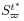. [554.1.2]Invariance of a measure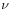on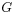under the induced dynamicsis defined as usual (see (3)) by requiring that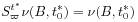(22)

for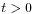and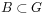. [554.1.3]For(22) may be called the condition of fractional invariance or fractional stationarity. [554.1.4]Using (2) the invariance condition becomes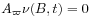(23)

[page 555, §0]   forwhere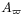is the infinitesimal generator of the semigroup. [555.0.1]For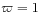the relation (21) implies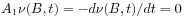, and thus in this case invariant measures conserve volumes in phase space as usual. [555.0.2]A very different situation arises for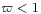.

[555.1.1]Forthe infinitesimal generators of the stable convolution semigroupare obtained  by evaluating the generalized function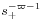on the time translation group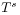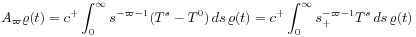(24)

where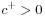is a constant. [555.1.2]Comparing (24) with the Balakrishnan algorithm [30, 31, 32] for fractional powers of the generator of a semigroup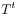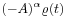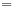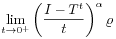(25)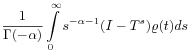shows that if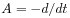denotes the infinitesimal generator of the original time evolutionthen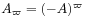is the infinitesimal generator of the induced time evolution. [555.1.3]Forthe generatorsfor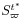are fractional time derivatives [15, 31, 29]. [555.1.4]The differential form (23) of the fractional invariance condition forbecomes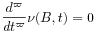(26)

forwhich was first derived in [18, 19]. [555.1.5]Its solution is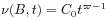(27)

forwith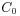a constant. [555.1.6]This shows that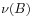for a fractional stationary dynamical state is not constant. [555.1.7]Fractional stationarity or fractional invariance of a measureimplies that phase space volumesshrink with time. [555.1.8]Thus fractional dynamics is dissipative. [555.1.9]More generally (26) reads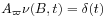with solution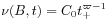for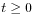in the sense of distributions. [555.1.10]The stationary solution withhas a jump discontinuity at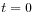, and is not simply constant.

[555.2.1]The transition from an original invariant measure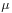on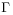to a fractional invariant measureon a subsetof measure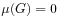may be called stationarity breaking. [555.2.2]It occurs spontaneously in the sense that it is generated by the dynamics itself. [555.2.3]Stationarity breaking implies ergodicity breaking, and thus the ultralong time limit is a possible scenario for ergodicity breaking in ergodic theory.

[555.3.1]The present paper has shown that the use of fractional time derivatives in physics is not only justified, but arises generically for induced dynamics in the ultralong time limit. [555.3.2]This mathematical result applies to many physical situations. [555.3.3]In the simplest case the resulting fractional differential equation (26) defines fractional stationarity which provides the dynamical basis for the anequilibrium concept . [555.3.4]Recently fractional random walks were discussed  and solved  in the continuum limit.

[page 556, §0]   ACKNOWLEDGEMENT : The author is grateful to Norges Forskningsrad (Nr.: 424.94 / 004 B) for financial support.